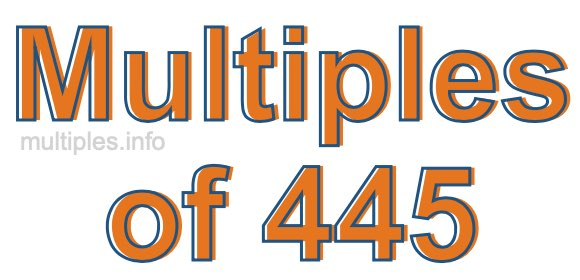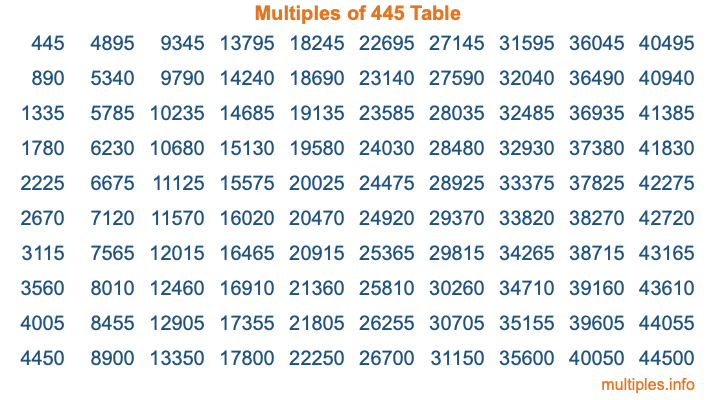Multiples of 445Welcome to the Multiples of 445 page. Here we will first teach you everything you will ever need to know about the multiples of 445, and then give you a study guide summary of everything we taught you to make sure you remember it all. Use this page to look up facts and learn information about the multiples of 445. This page will make you a multiples of four hundred forty-five expert!

Definition of Multiples of 445
Multiples of 445 are all the numbers that when divided by 445 equal an integer. Each of the multiples of 445 are called a multiple. A multiple of 445 is created by multiplying 445 by an integer.

Therefore, to create a list of multiples of 445, you start with 1 multiplied by 445, then 2 multiplied by 445, then 3 multiplied by 445, and so on for as long as you want. Thus, the list of the first five multiples of 445 is 445, 890, 1335, 1780, and 2225. To see a larger list of multiples of 445, see the printable image of Multiples of 445 further down on this page. We also have a category where you can choose any nth multiple of 445.

Multiples of 445 Checker
The Multiples of 445 Checker below checks to see if any number of your choice is a multiple of 445. In other words, it checks to see if there is any number (integer) that when multiplied by 445 will equal your number. To do that, we divide your number by 445. If the the quotient is an integer, then your number is a multiple of 445.

Is  a multiple of 445?

Least Common Multiple of 445 and ...
A Least Common Multiple (LCM) is the lowest multiple that two or more numbers have in common. This is also called the smallest common multiple or lowest common multiple and is useful to know when you are adding our subtracting fractions. Enter one or more numbers below (445 is already entered) to find the LCM.

Check out our LCM Calculator if you need more details about the Least Common Multiple or if you need the LCM for different numbers for adding and subtraction fractions.

nth Multiple of 445
As we stated above, 445 is the first multiple of 445, 890 is the second multiple of 445, 1335 is the third multiple of 445, and so on. Enter a number below to find the nth multiple of 445.

th multiple of 445

Multiples of 445 vs Factors of 445
445 is a multiple of 445 and a factor of 445, but that is where the similarities end. All postive multiples of 445 are 445 or greater than 445. All positive factors of 445 are 445 or less than 445.

Below is the beginning list of multiples of 445 and the factors of 445 so you can compare:

Multiples of 445: 445, 890, 1335, 1780, 2225, etc.

Factors of 445: 1, 5, 89, 445

As you can see, the multiples of 445 are all the numbers that you can divide by 445 to get a whole number. The factors of 445, on the other hand, are all the whole numbers that you can multiply by another whole number to get 445.

It's also interesting to note that if a number (x) is a factor of 445, then 445 will also be a multiple of that number (x).

Multiples of 445 vs Divisors of 445
The divisors of 445 are all the integers that 445 can be divided by evenly. Below is a list of the divisors of 445.

Divisors of 445: 1, 5, 89, 445

The interesting thing to note here is that if you take any multiple of 445 and divide it by a divisor of 445, you will see that the quotient is an integer.

Multiples of 445 Table
Below is an image of the first 100 multiples of 445 in a table. The table is in chronological order, column by column. The first column has the first ten multiples of 445, the second column has the next ten multiples of 445, and so on.The Multiples of 445 Table is also referred to as the 445 Times Table or Times Table of 445. You are welcome to print out our table for your studies.

Negative Multiples of 445
Although not often discussed or needed in math, it is worth mentioning that you can make a list of negative multiples of 445 by multiplying 445 by -1, then by -2, then by -3, and so on, to get the following list of negative multiples of 445:

-445, -890, -1335, -1780, -2225, etc.

Multiples of 445 Summary
Below is a summary of important Multiples of 445 facts that we have discussed on this page. To retain the knowledge on this page, we recommend that you read through the summary and explain to yourself or a study partner why they hold true.

There are an infinite number of multiples of 445.

A multiple of 445 divided by 445 will equal a whole number.

445 divided by a factor of 445 equals a divisor of 445.

The nth multiple of 445 is n times 445.

The largest factor of 445 is equal to the first positive multiple of 445.

445 is a multiple of every factor of 445.

445 is a multiple of 445.

A multiple of 445 divided by a divisor of 445 equals an integer.

445 divided by a divisor of 445 equals a factor of 445.

Any integer times 445 will equal a multiple of 445.

Multiples of a Number
Here you can get the multiples of another number, all with the same attention to detail as we did for multiples of 445 on this page.

Multiples of
Multiples of 446
Did you find our page about multiples of four hundred forty-five educational? Do you want more knowledge? Check out the multiples of the next number on our list!